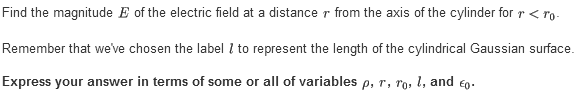# Finding magnitude of electric field of a cylinder

ecsx00

## Homework Statement## Homework Equations

E = 1/4($\pi$ $\epsilon_{0}$) * $\frac{p}{r^2}$

## The Attempt at a Solution

E = 2$\pi$$r_{0}$ $\epsilon_{0}$ = pl/$\epsilon_{0}$
= $\frac{pr}{2\pi r_{0} \epsilon_{0}}$

I am going by what I know about Gauss Law and using a similar format for the Electric field equation for a infinite charge line in a cylinder.

I fixed it little by little and I left off at:
E = $\frac{r}{2 \epsilon_{0}}$
The hint it gives me is that i am missing p but putting p in the numerator or denominator will say it is not dependent on p.
I probably did something wrong in the process or used a wrong equation.

Muphrid
What you have written is gibberish. Is this a uniformly charged cylinder?

You have to start at Gauss's law:

$$\oint E \cdot dA = Q_\text{enc}/\epsilon_0$$

And then invoke cylindrical symmetry to say that

$$|E|A = Q_\text{enc}/\epsilon_0$$

What is the area $A$ of the Gaussian surface? What is the charge enclosed by this surface?